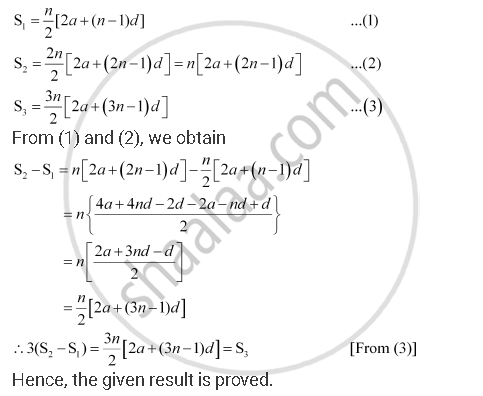CBSE (Arts) Class 11CBSE
Share

Books Shortlist

Let the Sum of N, 2n, 3n Terms of an Arithmetic Progression. Be S1, S2 and S3, Respectively, Show that S3 = 3 (S2– S1) - CBSE (Arts) Class 11 - Mathematics

ConceptArithmetic Progression (A.P.)

Question

Let the sum of n, 2n, 3n terms of an A.P. be S1, S2 and S3, respectively, show that S3 = 3 (S2– S1)

Solution

Let a and b be the first term and the common difference of the A.P. respectively.

Therefore,Is there an error in this question or solution?

APPEARS IN

NCERT Solution for Mathematics Textbook for Class 11 (2013 to Current)
Chapter 9: Sequences and Series
Q: 3 | Page no. 199
Solution Let the Sum of N, 2n, 3n Terms of an Arithmetic Progression. Be S1, S2 and S3, Respectively, Show that S3 = 3 (S2– S1) Concept: Arithmetic Progression (A.P.).
S